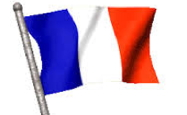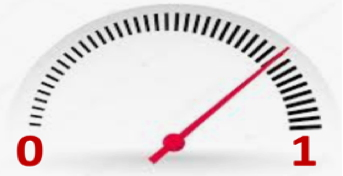highlight / actuality

# The war of quantum coherence​A quantum computer is the meeting point of two very different fields of research: On the one hand, physicists, on the other hand, researchers in theoretical computing. When these two worlds meet, the conclusions are unexpected.

Published on 19 April 2019

A quantum computer is the meeting point of two very different fields of research. On the one hand, physicists who, over the past twenty years or so, have been able to control increasingly complex quantum states; this is the second quantum revolution. On the other hand, researchers in theoretical computing are imagining a new type of calculation that would exploit quantum mechanics to obtain performances unimaginable with existing computers. Today, a major effort is deployed to try to bring these two worlds together.

The quantum bit that generalizes the 0 and 1 of our computers is a continuous object: it is described by a small needle that can point continuously between 0 and 1. The supposed power of quantum computers comes from a phenomenon called entanglement: if a single needle is sufficient to describe a quantum bit, an exponential number 2N is needed to describe the state of a machine with N quantum bits. Thus, the state of a machine with only 30 quantum bits is described by more than a billion small needles. This proliferation of degrees of freedom would make it possible to carry out massively parallel calculations.But entanglement is also the nightmare of the quantum computer because it is necessary to be able to control precisely all these small needles while avoiding them to be disrupted by the other degrees of freedom present around them (vibration of atoms, fluctuations of the electromagnetic field...).

In an attempt to control this precision problem, theoretical computer researchers have envisioned "quantum error correction codes" where many quantum bits are used to form a single quantum metabit.

In recent work, Xavier Waintal, a researcher at IRIG's Quantum Photonics, Electronics and Engineering Laboratory, has examined these codes from the point of view of a physicist. His conclusions are rather pessimistic: he shows that a great many orders of magnitude stand between what we can achieve and what would be needed to envision building these quantum technologies.

In quantum computing, a quantum bit or qubit is the quantum state that represents the smallest unit of quantum information storage. A qubit memory differs significantly from a conventional memory in that one bit can only take the values 0 and 1, and only one at a time. A qubit does not have this restriction.
In quantum mechanics, quantum entanglement is a phenomenon in which two particles form a linked system and present quantum states that depend on each other regardless of the distance between them.
A quantum error correction (QEC) code uses several quantum bits to construct a "logical" quantum bit of better quality than the initial "physical" bit.

Top page